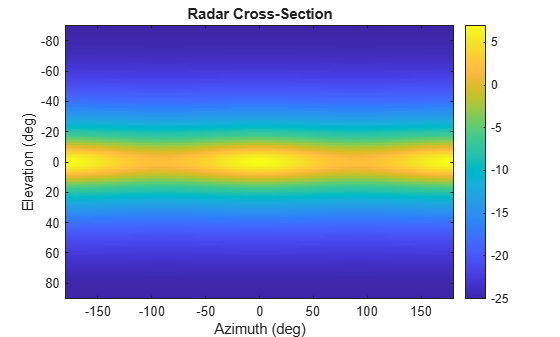# rcsSignature

## Description

`rcsSignature` creates a radar cross-section (RCS) signature object. You can use this object to model an angle-dependent and frequency-dependent radar cross-section pattern. The radar cross-section determines the intensity of reflected radar signal power from a target. The object models only non-polarized signals.

## Creation

### Syntax

``rcssig = rcsSignature``
``rcssig = rcsSignature(Name,Value)``

### Description

``` `rcssig = rcsSignature` creates an `rcsSignature` object with default property values.```

example

````rcssig = rcsSignature(Name,Value)` sets object properties using one or more `Name,Value` pair arguments. `Name` is a property name and `Value` is the corresponding value. `Name` must appear inside single quotes (`''`). You can specify several name-value pair arguments in any order as `Name1,Value1,...,NameN,ValueN`. Any unspecified properties take default values.```

Note

You can only set property values of `rcsSignature` when constructing the object. The property values are not changeable after construction.

## Properties

expand all

Sampled radar cross-section (RCS) pattern, specified as a scalar, a Q-by-P real-valued matrix, or a Q-by-P-by-K real-valued array. The pattern is an array of RCS values defined on a grid of elevation angles, azimuth angles, and frequencies. Azimuth and elevation are defined in the body frame of the target.

• Q is the number of RCS samples in elevation.

• P is the number of RCS samples in azimuth.

• K is the number of RCS samples in frequency.

Q, P, and K usually match the length of the vectors defined in the `Elevation`, `Azimuth`, and `Frequency` properties, respectively, with these exceptions:

• To model an RCS pattern for an elevation cut (constant azimuth), you can specify the RCS pattern as a Q-by-1 vector or a 1-by-Q-by-K matrix. Then, the elevation vector specified in the `Elevation` property must have length 2.

• To model an RCS pattern for an azimuth cut (constant elevation), you can specify the RCS pattern as a 1-by-P vector or a 1-by-P-by-K matrix. Then, the azimuth vector specified in the `Azimuth` property must have length 2.

• To model an RCS pattern for one frequency, you can specify the RCS pattern as a Q-by-P matrix. Then, the frequency vector specified in the `Frequency` property must have length 2.

Example: `[10,0;0,-5]`

Data Types: `double`

Azimuth angles used to define the angular coordinates of each column of the matrix or array, specified by the `Pattern` property. Specify the azimuth angles as a length-P vector. P must be greater than two. Angle units are in degrees.

Example: `[-45:0.5:45]`

Data Types: `double`

Elevation angles used to define the coordinates of each row of the matrix or array, specified by the `Pattern` property. Specify the elevation angles as a length-Q vector. Q must be greater than two. Angle units are in degrees.

Example: `[-30:0.5:30]`

Data Types: `double`

Frequencies used to define the applicable RCS for each page of the `Pattern` property, specified as a K-element vector of positive scalars. K is the number of RCS samples in frequency. K must be no less than two. Frequency units are in hertz.

Example: `[0:0.1:30]`

Data Types: `double`

## Object Functions

 `value` Radar cross-section at specified angle and frequency `toStruct` Convert to structure

## Examples

collapse all

Specify the radar cross-section (RCS) of a triaxial ellipsoid and plot RCS values along an azimuth cut.

Specify the lengths of the axes of the ellipsoid. Units are in meters.

```a = 0.15; b = 0.20; c = 0.95;```

Create an RCS array. Specify the range of azimuth and elevation angles over which RCS is defined. Then, use an analytical model to compute the radar cross-section of the ellipsoid. Create an image of the RCS.

```az = [-180:1:180]; el = [-90:1:90]; rcs = rcs_ellipsoid(a,b,c,az,el); rcsdb = 10*log10(rcs); imagesc(az,el,rcsdb) title('Radar Cross-Section') xlabel('Azimuth (deg)') ylabel('Elevation (deg)') colorbar```Create an `rcsSignature` object and plot an elevation cut at $3{0}^{\circ }$ azimuth.

```rcssig = rcsSignature('Pattern',rcsdb,'Azimuth',az,'Elevation',el,'Frequency',[300e6 300e6]); rcsdb1 = value(rcssig,30,el,300e6); plot(el,rcsdb1) grid title('Elevation Profile of Radar Cross-Section') xlabel('Elevation (deg)') ylabel('RCS (dBsm)')``````function rcs = rcs_ellipsoid(a,b,c,az,el) sinaz = sind(az); cosaz = cosd(az); sintheta = sind(90 - el); costheta = cosd(90 - el); denom = (a^2*(sintheta'.^2)*cosaz.^2 + b^2*(sintheta'.^2)*sinaz.^2 + c^2*(costheta'.^2)*ones(size(cosaz))).^2; rcs = (pi*a^2*b^2*c^2)./denom; end```

 Richards, Mark A. Fundamentals of Radar Signal Processing. New York, McGraw-Hill, 2005.